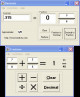Abilities Builder Add & Subtract 6.6 Improve whole number computation skills from addition through division. DemoAbilities Builder Add & Subtract Whole Numbers 6.1 Improve whole number addition and subtraction computation skills. DemoMS Access Add, Subtract, Multiply, Divide Fields S 7.0 Apply math to fields (columns) in MS Access tables. SharewareBreaktru Fractions n Decimals 10.2 Add, Subtract, Divide and Multiply fractions. Convert decimals / fractions SharewareFrakKalk 2.0 FrakKalk allows you to add, subtract, multiply, and divide large fractions without having to convert from decimal form. FreewareAdjust Indents 1.0 The Adjust Indents package, for Tex-Edit Plus, contains two scripts (Tab add and Tab subtract). FreewareList Remove, Compare & Duplicate Manager Software 7.0 List manager; delete duplicates from the list, subtract one list from another... SharewareFRS Money Math X 1.8 FRS Money Math is a fun and easy to use tool to aid students in learning to identify, total, add, and subtract money. SharewareFractions n Decimals CE 5.3 Add, Subtract, Divide and Multiply fractions. Convert decimals/fractions CE 2.11 SharewareDTP Multicalc 1.3 DTP Multicalc calculates picas, points, inches and cms/mms. Freeware